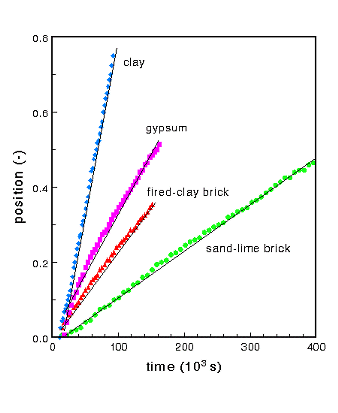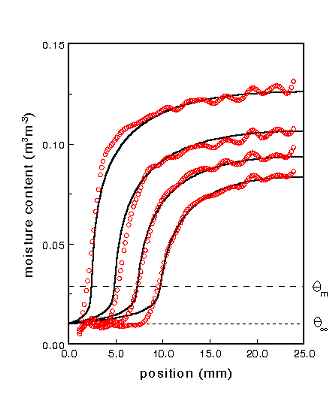ANALYTIC SOLUTION
for the non-linear drying problem

In engineering applications one prefers a simple, but adequate description, which simulates the drying of a material correctly. It is usual for the moisture transport to be described by a non-linear diffusion model:In this equation D is the moisture diffusivity. In this 'lumped' model all mechanisms for moisture transport, i.e., liquid flow and vapour diffusion, are combined into a single moisture diffusivity, which is dependent on the actual moisture content.  Until now, numerical simulations have been the only way to solve the non-linear diffusion equation. However, numerical simulations provide no basic understanding of the drying process. Recently we proposed an analytic solution for a non-linear drying problem using a quasi-steady state solution. An important consequence of this model is that the drying front has a constant speed when it is entering the material.  This has also been observed in drying experiments as is illustrated in figure 1 .Figure 1:  The dimensionless position of the drying front for various drying experiments:
clay ,  gypsum,  fired-clay brick  and  sand-lime brick.

In figure 2 an examples is given of moisture profiles as measured for gypsum. The profiles determined by the fitted analytic model are also plotted in Fig. 2. As can be seen the analytic model describes the moisture profiles especially well for the liquid part of the moisture profile. The drying front is also reproduced very nicely. For all materials the analytic model overestimates the moisture content in the vapour range.Figure 2: The measured moisture concentration profiles after 10, 20, 30 and 40 hours of  drying as measured by NMR. The solid lines give the moisture profiles as determined by the analytic model.

• An extensive description of this model can be found in:
• K.A. Landman, L.Pel, E.F. Kaasschieter, Analytic modelling of drying of porous materials, Mathematical Engineering in Industry 8, 89-122 (2001).

L. Pel, K.A. Landman, and E.F. Kaasschieter, Analytic solution for the non-linear drying problem, Int. J. Heat Mass Transfer 45, 3174-3180 (2002).

L. Pel, E.F. Kaasschieter and K.A. Landman, A sharp drying front model, Drying technology 22, 637-647 (2004).